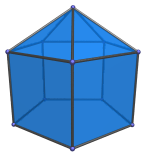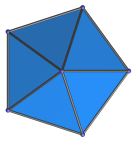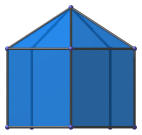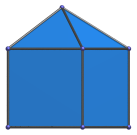# The Elongated Pentagonal Pyramid

The elongated pentagonal pyramid is the 9th Johnson solid (J9). It has 11 vertices, 20 edges, and 11 faces (5 equilateral triangles, 5 squares, and 1 pentagon).The elongated pentagonal pyramid can be constructed by attaching a pentagonal pyramid to a pentagonal prism.

Attaching a second pentagonal pyramid to the other end of the pentagonal prism produces the elongated pentagonal bipyramid (J16).

## Projections

Here are some views of the elongated pentagonal pyramid from various angles:

Projection Envelope DescriptionRegular pentagon

Top view.Pentagon

Side view.Pentagon

Front view.

## Coordinates

The Cartesian coordinates of the elongated pentagonal pyramid with edge length 2 are:

• (0, 0, 1+√((10−2√5)/5))
• (√((10+2√5)/5), 0, ±1)
• (√((5−√5)/10), ±φ, ±1)
• (−√((5+2√5)/5), ±1, ±1)

where φ=(1+√5)/2 is the Golden Ratio.

Last updated 18 Jun 2019.